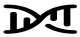# Instrumental variable (IV)

An IV is a variable (characteristic) that is robustly associated with the risk factor of interest and can be used to explore the unconfounded causal effect of that risk factor on an outcome, under the assumptions described here.

There are three core assumptions of IV analyses: 1. The IV is robustly associated with the risk factor of interest (i.e., replication of its association has been demonstrated). In econometrics, this is known as the relevance assumption. The IV is not associated with confounders of the risk factor-outcome association. In econometrics, this is known as the independence or exchangeability assumption. The IV is only associated with the outcome through the risk factor. In econometrics, this is known as the exclusion restriction assumption. Additional assumptions are required to identify a well-defined causal parameter. These assumptions can be grouped as follows: - Homogeneity assumptions: assuming either that the association between the IV and the exposure is homogeneous (i.e., is the same for everyone in the population) or that the effect of the exposure on the outcome is homogeneous. If true, this implies that the IV estimate is consistent with the average causal effect (ACE). - No effect modification: if the IV does not modify the effect of the exposure on the outcome within levels of the exposure and for all levels of the exposure, then the IV estimate is consistent with the ACE even if the effect of the exposure on the outcome is heterogeneous. - Deterministic Monotonicity: often referred to simply as “monotonicity”, this assumes a monotonic relationship between the IV and exposure. In other words, a (genetic) IV could not increase the exposure in some people and decrease it in others. This allows the assumption that the effect of the IV on the exposure is homogeneous to be relaxed. If monotonicity holds, then the IV estimate is consistent with the ACE among compliers (under deterministic monotonicity, this corresponds to the subgroup of the sample affected by the IV). This causal estimate does not straightforwardly apply to continuous exposures. - Stochastic Monotonicity: this is a relaxation of deterministic monotonicity, because this only requires that a monotonic increasing association between the IV and the exposure exists conditionally on a set of covariates (which may or may not be measured). If this holds, then the IV estimate is consistent for a weighted average of treatment effects, such that more weight is given to treatment effects among subgroups where the effect of the IV on the exposure is greater. This assumption applies to both binary and continuous exposures but allows identifying an estimate that is less useful and harder to clearly define than in the ACE among compliers.